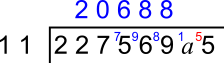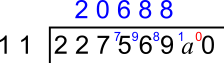#### You may also like### Consecutive Numbers

An investigation involving adding and subtracting sets of consecutive numbers. Lots to find out, lots to explore.### Have You Got It?

Can you explain the strategy for winning this game with any target?### Counting Factors

Is there an efficient way to work out how many factors a large number has?

# Divisible by 55

##### Age 11 to 14 ShortChallenge Level

Answer: $a$ = 6 and $b = 5$ or $a$ = 1 and $b$ = 0

Multiples of 5 and 11
55 = 5$\times$11

227569$ab$ is a multiple of 5 $\therefore b$ must be 5 or 0.

If $b$ = 5,remainder when 1$a$ is divided by 11 must be 5
$\therefore a$ = 6

If $b$ = 0,remainder when 1$a$ is divided by 11 must be 0
$\therefore a$ = 1

Considering multiples of 55
We can look for multiples of 55 that are close to 22756900

Divide by 55/find a nearby multiple
55 $\times$ 4 = 220          22000000 is a multiple of 55
+     550000
22550000 is also a multiple of 55
55 $\times$ 3 = 165      +     165000
22715000
55 $\times$ 5 = 275       +      27500
22742500
55 $\times$ 2 = 110       +      11000
22753500
55 $\times$ 6 = 330       +        3300
22756800

Close multiples of 55:
22756800
22756855
22756910 ($a$ = 1 and $b$ = 0)
22756965 ($a$ = 6 and $b$ = 5)

You can find more short problems, arranged by curriculum topic, in our short problems collection.